# Magnetic Fields and Forces Flashcards

Set Details Share
created 5 years ago by ssdesa_100
1,843 views
updated 5 years ago by ssdesa_100
Page to share:
Embed this setcancel
COPY
code changes based on your size selection
Size:
X

1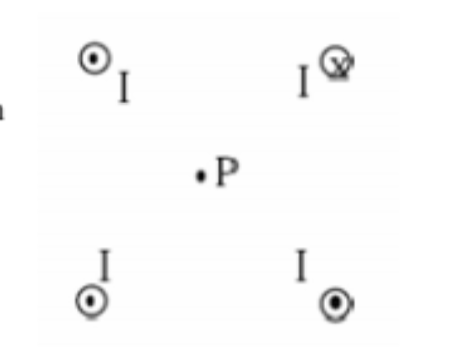Four infinitely long wires are arranged as shown in the accompanying figure end–on view. All four wires are perpendicular to the plane of the page and have the same magnitude of current I. The conventional current in the wire in the upper right–hand corner is directed into the plane of the page. The other conventional currents are out of the plan of the page. Point P is a distance a from all four wires. What is the total magnetic field at point P?

(A) (

(C)

2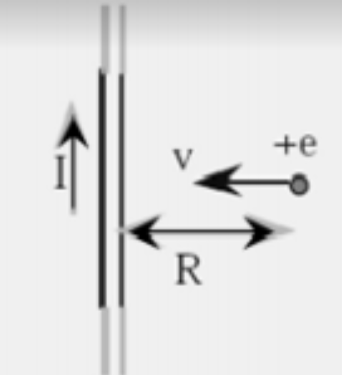The conventional current I in a long straight wire flows in the upward direction as shown in the figure. (Electron flow is downward.) At the instant a proton of charge +e is a distance R from the wire and heading directly toward it, the force on the proton is:

(A) (

(E)

3

A charged particle with constant speed enters a uniform magnetic field whose direction is perpendicular to the particles velocity. The particle will:

(A) Speed up

(B) Experience no change in velocity

(E)

4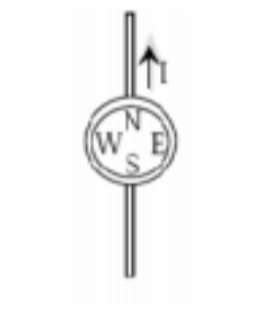A long straight wire conductor is placed below a compass as shown in the top view figure. When a large conventional current flows in the conductor as shown, the N pole of the compass:

(A) has its polarity reversed

(B) points to the south

(C) points to the west

(D) points to the east

(D)

5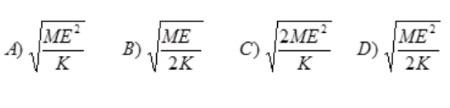A proton of mass M and kinetic energy K passes undeflected through a region with electric and magnetic fields perpendicular to each other. The electric field has magnitude E. The magnitude of the magnetic field B is:

(A)
(B)
(C)
(D)

(D)

6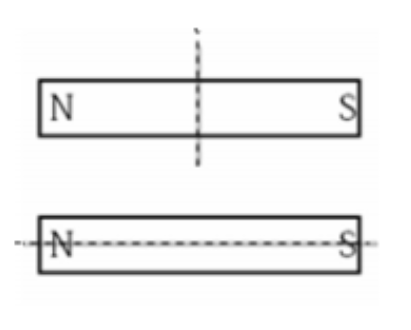Two bar magnets are to be cut in half along the dotted lines shown. None of the pieces are rotated. After the cut:

(A) The two halves of each magnet will attract each other

(B) The two halves of each magnet will repel each other

(C) The two halves of the top magnet will repel, the two halves of the
bottom magnet will attract

(D) The two halves of the top magnet will attract, the two halves of the bottom magnet will repel

(A)

7

An ion with charge q, mass m, and speed v enters a magnetic field B and is deflected into a path with a radius of curvature R. If a second ion has speed 2v, while m, q, and B are unchanged, what will be the radius of the second ion’s path?

(A) 4R

(B) 2R

(C) R/2

(D) R/4

(E)

8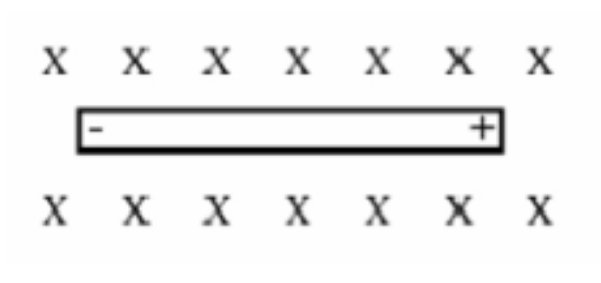A wire moves through a magnetic field directed into the page. The wire experiences an induced charge separation as shown. Which way is the wire moving?

(A) to the right

(B) to the left

(C) toward the top of the page

(D) toward the bottom of the page

(B)

9

A charged particle with constant velocity enters a uniform magnetic field whose direction is parallel to the particle’s velocity. The particle will:

(A) speed up

(B) slow down

(C) experience no change in velocity

(E)

10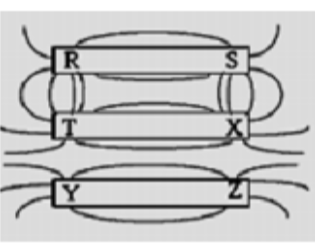The diagram to the right depicts iron filings sprinkled around three
permanent magnets. Pole R is the same pole as:
A) T and Y
B) T and Z
C) X and Y
D) X and Z

(C)

11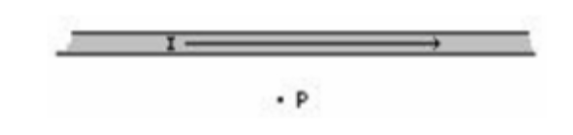If conventional electric current flows from left to right in a wire as shown, what is the direction of the magnetic field at point P?
A) towards the top of the paper
B) towards the bottom of the paper
C) into the paper
D) out of the paper

(D)

12

Two light wires are hung vertically. With electrical current in both wires directed upwards
A) the wires will experience a force of attraction
B) the wires will experience a force of repulsion
C) the force on the right hand wire will cancel the force on the left hand wire
D) both wires will experience a torque until they are at right angles to each other

(C)

13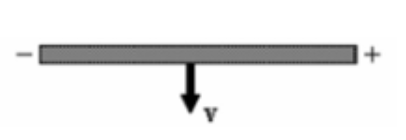A wire moves with a velocity v through a magnetic field and
experiences an induced charge separation as shown. What is the
direction of the magnetic field?
A) into the page
B) towards the bottom of the page
C) out of the page
D) towards the top of the page

(A)

14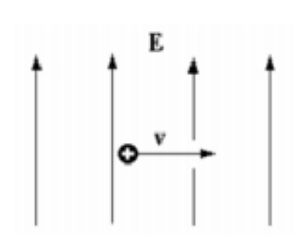A positively charged particle moves to the right. It enters a region of space in which there is an electric field directed up the plane of the paper as shown. In which direction does the magnetic field have to point in this region so that the:
particle maintains a constant velocity?
A) into the plane of the page
B) out of the plane of the page
C) to the right
D) to the left

(A)

15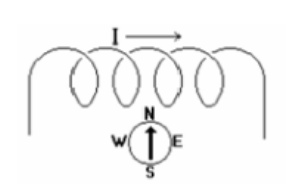A compass is placed near a coil of wire. A conventional electrical
current is then run through the coil from left to right as shown. This
will cause the North pole of the compass to:
A) point toward the left
B) point toward the right
C) point toward the bottom of the paper
D) not move since the magnetic field of the coil is into the paper

(B)

16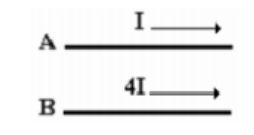Two parallel wires are carrying different electric current in the same direction as shown. How does the magnitude of the force of A from B compare to the force of B from A?

(A) FB on A = 1/4FA on B

(B) FB on A = 2FA on B

(C) FB on A = 1/2FA on B

(D) FB on A = FA on B

(A)

17

A positively charged particle of mass M is at rest on a table. A non–zero electric field E is directed into the plane of the table. A non–zero magnetic field B is directed out of the plane of the table. What is true about the magnitude of the electric force on the particle FE compared to the magnetic force on the particle FB?

(A) FE > FB

(B) FE < FB

(C) FE = FB

(D) It cannot be determined without knowing the exact value of the charge of the particle.

(E)

18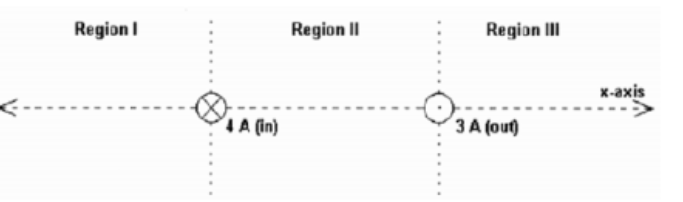Two very long current–carrying wires are shown end on in the figure. The wire on the left has a 4A current going into the plane of the paper and the wire on the right has a 3A current coming out of the paper. Disregarding the case of x ∞, in which region(s) could the magnetic field from these two wires add to zero
on the x–axis?

(A) Region I only

(B) Region II only

(C) Region III only

(D) Regions I and III only

(A)

19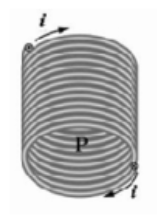The magnetic field line passing through point P inside the solenoid is directed
A) to the right
B) to the left
C) downward toward the bottom of the page
D) upward toward the top of the page

(E)

20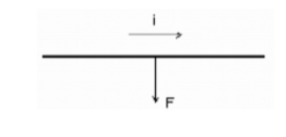The diagram below shows a straight wire carrying a current i in a uniform magnetic field. An arrow indicates the magnetic force F on the wire. Of the following possibilities, the direction of the magnetic field must be:
A) out of the page
B) into the page
C) up the plane of the page
D) down the plane of the page

(C)

21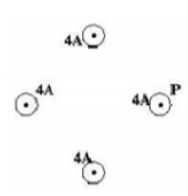For the four identical current-carrying wires shown (with conventional
current coming out of the plane of the page), the wire on the right is
labeled P. What is the direction of the total magnetic force on the wire labeled P that is caused by the other wires?
A) To the left
B) To the right
C) Towards the top of the page
D) There is no force.

(D)

22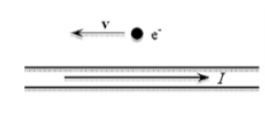A wire has a conventional current I directed to the right. At the instant shown in the figure, an electron has a velocity directed to the left. The magnetic force on the electron at this instant is:
A) directed toward the top of the page.
B) directed toward the bottom of the page.
C) directed out of the plane of the page.
D) directed into the plane of the page.

(A)

23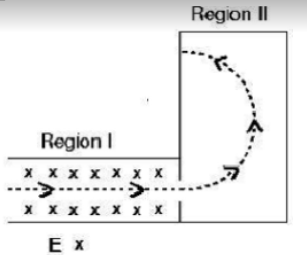An electron moves in the plane of the page through two
regions of space along the dotted-line trajectory shown in
the figure. There is a uniform electric field in Region I directed into the plane of the page (as shown). There is no electric field in Region II. What is a necessary direction of the magnetic field in regions I and II? Ignore gravitational forces.

Region I Region II

(A) Toward bottom of the page | Up on the page
(B) Toward top of the page | Into the page
(C) Toward top of the page | Out of the page
(D) Toward bottom of the page | Out of the page

(A)

24

A proton moves toward the top of this page into a region
that has a magnetic field directed to the right of this page. If the particle is undeflected as it passes through this region, in what direction must there be a component of electric field? Ignore gravity.

(A) To the left

(B) Into the page

(C) Out of the page

(D) To the right

(E)

25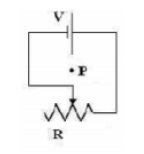For the figure shown, the variable resistance in the circuit is increased at a constant rate. What is the direction of the magnetic field at the point P at the center of the circuit?

Magnetic Field at P
(A) Into the page
(B) Out of the page
(C) To the left
(D) There is no field

(C)

26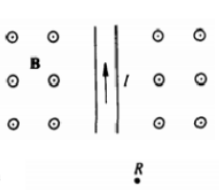A wire in the plane of the page carries a current directed toward the
top of the page as shown. If the wire is located in a uniform magnetic field B directed out of the page, the force on the wire resulting from the magnetic field is:
(A) directed to the left
(B) directed out of the page
(C) directed to the right
(D) zero

(C)

27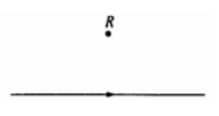The direction of the magnetic field at point R caused by the current I in the wire shown is:
(A) to the left

(B) to the right

(C) into the page

(D) out of the page

(A)

28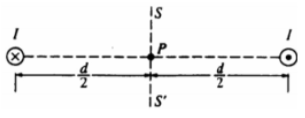Two long, parallel wires are separated by a distance d, as shown. One wire carries a steady current I into the plane of the page while the other wire carries a steady current I out of the page. At what points in the plane of the page and outside the wires, besides points at infinity, is the magnetic field due to the currents zero?
(A) Only at point P
(B) At all points on the line SS'
(C) At all points on the line connecting the two wires
(D) At no points

(D)

29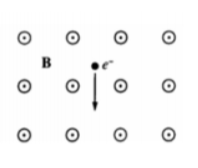An electron is in a uniform magnetic field B that is directed out of the plane of the page, as shown. When the electron is moving in the plane of the page in the direction indicated by the arrow, the force on the electron is directed:
(A) toward the right
(B) out of the page
(C) into the page
(D) toward the top of the page

(C)

30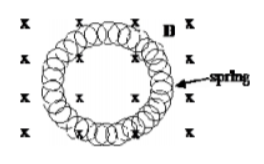A metal spring has its ends attached so that it forms a circle. It is
placed in a uniform magnetic field, as shown. Which of the following will not cause a current to be induced in the spring?
(A) Changing the magnitude of the magnetic field
(B) Rotating the spring about a diameter
(C) Moving the spring parallel to the magnetic field
(D) Moving the spring in and out of the
magnetic field

(E)

31

A magnetic field of 0.1T forces a proton beam of 1.5 mA to move in a circle of radius 0.1 m. The plane of the circle
is perpendicular to the magnetic field.

31.) Of the following, which is the best estimate of the work done by the magnetic field on the protons during one
complete orbit of the circle?
(A) 0 J (B) 10-22 J (C) 10-5 J (D) 102 J

(E)

32

A magnetic field of 0.1T forces a proton beam of 1.5 mA to move in a circle of radius 0.1 m. The plane of the circle
is perpendicular to the magnetic field.

32.) Of the following, which is the best estimate of the speed of a proton in the beam as it moves in the circle?
(A) 10-2 m/s (B) 103 m/s (C) 106 m/s (D) 108 m/s

(A)

33

Two parallel wires, each carrying a current I, repel each other with a force F. If both currents are doubled, the force of repulsion is:

(B) 2√2 F

(C) 4F

(C) 4√2 F

(E) 8F

(D)

34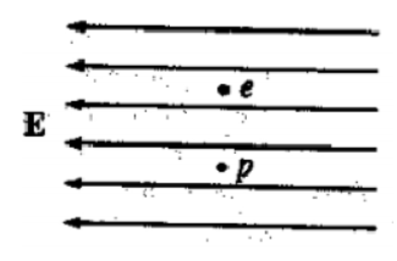An electron e and a proton p are simultaneously released from rest in a uniform electric field E, as shown. Assume that the particles are
sufficiently far apart so that the only force acting on each particle after it is released is that due to the electric field. At a later time when the particles are still in the field, the electron and the proton will have the same:
(A) speed
(B) displacement
(C) magnitude of acceleration
(D) magnitude of force acting on them

(A)

35

Two long, parallel wires, fixed in space, carry currents I1 and I2. The force of attraction has magnitude F. What
currents will give an attractive force of magnitude 4F?

(A) 2I1 and 1⁄2I2

(B) 1⁄2I1 and 1⁄2I2

(C) 2I1 and 2I2

(D) 4I1 and 4I2

(C)

36

A charged particle is projected with its initial velocity parallel to a uniform magnetic field. The resulting path is:

(A) a spiral

(B) a circular arc

(C) a straight line parallel to the field

(D) a straight line perpendicular to the field

(C)

37Two very long parallel wires carry equal currents in the same direction into the page, as shown. At point P, which is 10 centimeters from each wire, the magnetic field is:
A) zero
B) directed into the page
C) directed out of the page
D) directed to the right

(E)

38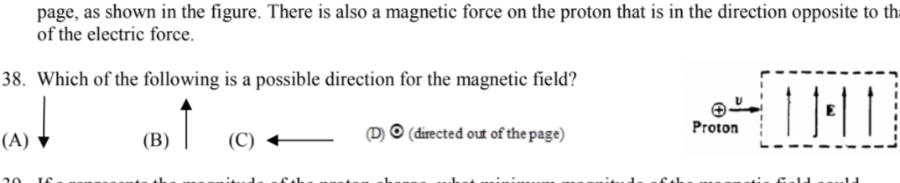A proton traveling with speed v enters a uniform electric field of magnitude E, directed parallel to the plane of the
page, as shown in the figure. There is also a magnetic force on the proton that is in the direction opposite to that
of the electric force.

Which of the following is a possible direction for the magnetic field?

(C)

39A proton traveling with speed v enters a uniform electric field of magnitude E, directed parallel to the plane of the
page, as shown in the figure. There is also a magnetic force on the proton that is in the direction opposite to that
of the electric force.

If e represents the magnitude of the proton charge, what minimum magnitude of the magnetic field could
balance the electric force on the proton?

(A) E/v

(B) eE/v

(C) vE

(D) eE

(D)

40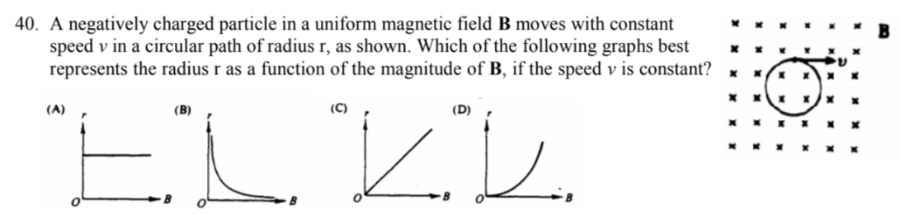A negatively charged particle in a uniform magnetic field B moves with constant speed v in a circular path of radius r, as shown. Which of the following graphs best represents the radius r as a function of the magnitude of B, if the speed v is constant?

(A)
(B)
(C)
(D)

(D)

41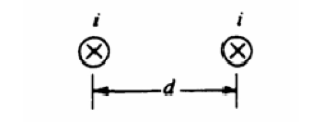Initially the wires are a distance d apart and each has a
current i directed into the page. The force per unit length on each wire has magnitude Fo.

The direction of the force on the right-hand wire due to the
current in the left-hand wire is:
(A) to the right
(B) to the left
(C) upward in the plane of the page
(D) downward in the plane of the page

(E)

42Initially the wires are a distance d apart and each has a
current i directed into the page. The force per unit length on each wire has magnitude Fo.

The wires are moved apart to a separation 2d and the current in each wire is increased to 2i. The new force per unit length on each wire is:

(A) Fo/4

(B) Fo/2

(C) Fo

(D) 2Fo

(D)

43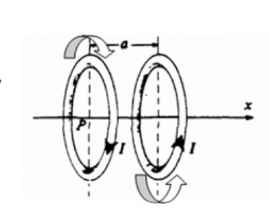Two identical parallel conducting rings have a common axis and are
separated by a distance a, as shown. The two rings each carry a current I, but in opposite directions. At point P, the center of the ring on the left the magnetic field due to these currents is:
(A) zero
(B) in the plane perpendicular to the x-axis
(C) directed in the positive x-direction
(D) directed in the negative x-direction

(A)

44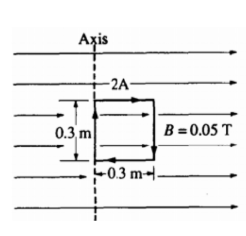A square loop of wire 0.3 meter on a side carries a current of 2 amperes and is located in a uniform 0.05-tesla magnetic field. The left side of the loop is aligned along and attached to a fixed axis. When the plane of the loop is parallel to the magnetic field in the position shown, what is the magnitude of the torque exerted on the loop about the axis?
A) 0.00225 Nm
B) 0.0090 Nm
C) 0.278 Nm
D) 1.11 Nm

(E)

45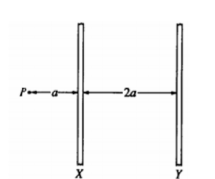Two long parallel wires are a distance 2a apart, as shown. Point P is in the plane of the wires and a distance a from wire X. When there is a current I in wire X and no current in wire Y, the magnitude of the magnetic field at P is Bo. When there are equal currents I in the same direction in both wires, the magnitude of the magnetic field at P is:

(A) 2Bo/3

(B) 10Bo/9

(C) 4Bo/3

(D) 2 Bo

(B)

46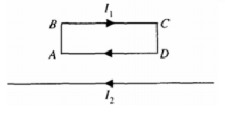A rigid, rectangular wire loop ABCD carrying current I1 lies in the plane of the page above a very long wire carrying current I2 as shown. The net force on the loop is:
(A) toward the very long wire
(B) away from the very long wire
(C) toward the left
(D) zero

(B)

47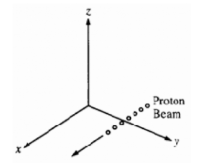A beam of protons moves parallel to the x-axis in the positive x-direction, as shown, through a region of crossed electric and magnetic fields balanced for zero deflection of the beam. If the magnetic field is pointed in the positive y-direction, in what direction must the electric field be pointed?
(A) Negative y-direction
(B) Positive z-direction
(C) Negative z-direction
(D) Negative x-direction

(D)

48

A charged particle can move with constant velocity through a region containing both an electric field and a magnetic field only if the:
(A) electric field is parallel to the magnetic field
(B) electric field is perpendicular to the magnetic field
(C) electric field is parallel to the velocity vector
(D) magnetic field is perpendicular to the velocity vector

(C)

49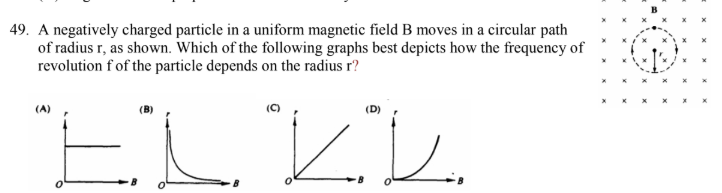A negatively charged particle in a uniform magnetic field B moves in a circular path of radius r, as shown. Which of the following graphs best depicts how the frequency of revolution f of the particle depends on the radius r?

(A)
(B)
(C)
(D)

(B)

50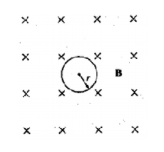A particle of charge +e and mass m moves with speed v perpendicular to a uniform magnetic field B directed into
the page. The path of the particle is a circle of radius r, as shown.

Which of the following correctly gives the direction of motion and the equation relating v and r ?

Direction Equation
(A) clockwise eBr = mv
(B) clockwise eBr = mv2
(C) counterclockwise eBr = mv
(D) counterclockwise eBr = mv2

(D)

51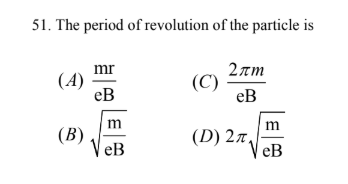A particle of charge +e and mass m moves with speed v perpendicular to a uniform magnetic field B directed into
the page. The path of the particle is a circle of radius r, as shown.

The period of revolution of the particle is:

(A)
(B)
(C)
(D)

(A)

52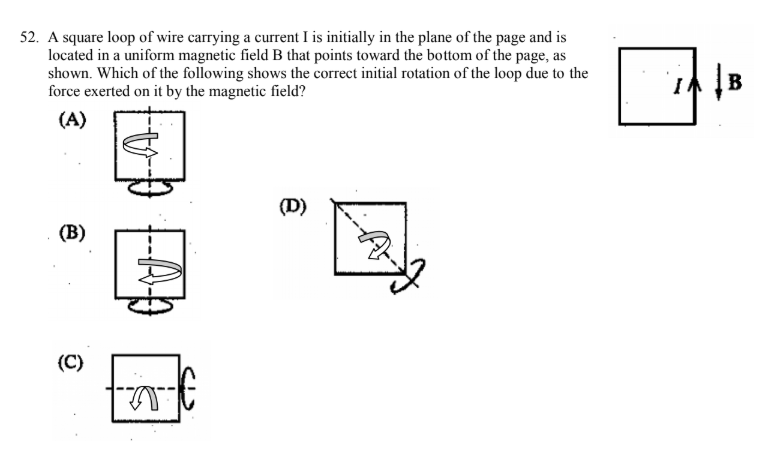A square loop of wire carrying a current I is initially in the plane of the page and is located in a uniform magnetic field B that points toward the bottom of the page, as shown. Which of the following shows the correct initial rotation of the loop due to the force exerted on it by the magnetic field?

(A)
(B)
(C)
(D)

(E)

53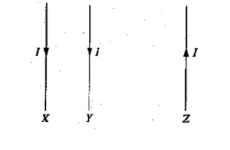The currents in three parallel wires, X, Y, and Z, each have magnitude I and are in the directions shown. Wire y is closer to wire X than to wire z. The magnetic force on wire y is:
(A) zero
(B) into the page
(C) out of the page
(D) toward the left

(B)

54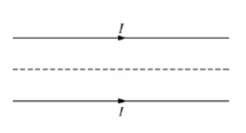Two long, straight, parallel wires in the plane of the page carry equal
currents I in the same direction, as shown above. Which of the
following correctly describes the forces acting on the wires and the
resultant magnetic field at points along the dotted line midway
between the wires?

Forces Field
(A) Attractive Not Zero
(B) Attractive Zero
(C) Repulsive Not Zero
(D) Repulsive Zero

(A)